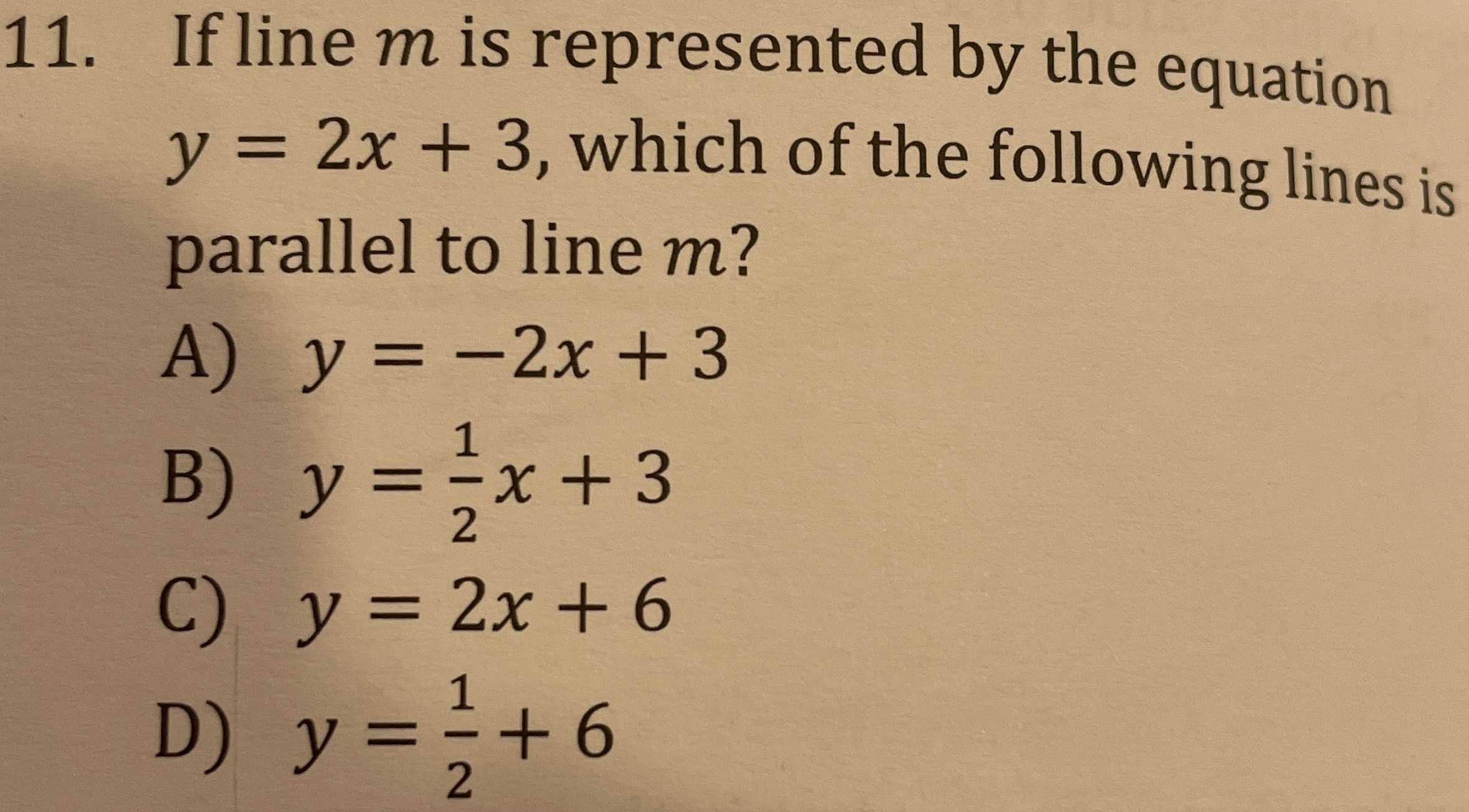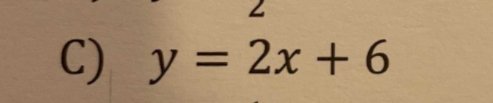### ¿Todavía tienes preguntas de matemáticas?

Pregunte a nuestros tutores expertos
Algebra
Pregunta11. If line $$m$$ is represented by the equation $$y = 2 x + 3$$ , which of the following lines is parallel to line $$m$$ ?

A) $$y = - 2 x + 3$$

B) $$y = \frac { 1 } { 2 } x + 3$$

C) $$y = 2 x + 6$$

D) $$y = \frac { 1 } { 2 } + 6$$